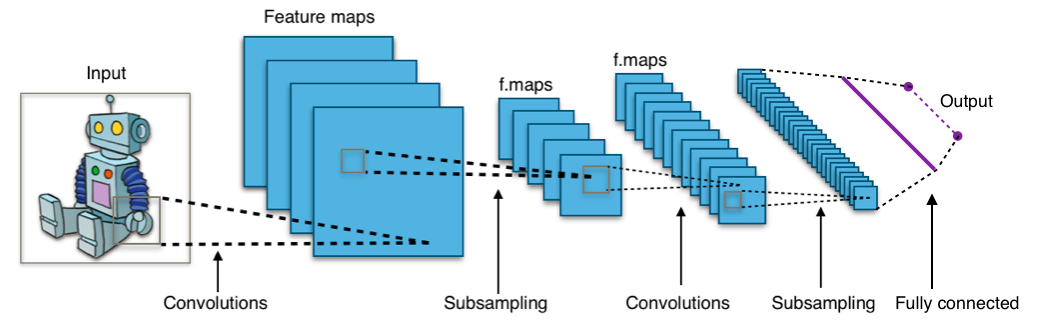# Introduction to deep learning

In this course, which consists of four chapters, you will learn to work with artificial neural networks using Keras, a Python library for deep learning. You will learn how to build and train a small neural network from scratch, how to use large, pretrained neural networks, and how to modify pretrained networks for your own purpose.

But before we dive into the code, let's introduce the basic concepts and terminology of deep learning!Figure 1. Your mysterious octopus teacher

## Concepts and terminology

### Artificial neural networks

Brains are biological neural networks. A brain consists of about 100 billion brain cells, or neurons, which are connected to each other through about 100 trillion synapses. When a neuron becomes active, it sends a signal to all other neurons to which it is connected. In response, some of these other neurons become active themselves and send signals to yet other neurons. And so on, in a cascade of neural activity that, in a way that neuroscientists are only just beginning to understand (but still don't, really), gives rise to feelings and thoughts.

An artificial neural network is a computer program that is inspired by biological neural networks. Artifical neural networks also consist of neurons (sometimes called nodes in this context) that are connected to each other. But artificial neurons and connections are software rather than cells.Figure 2. A simple artificial neural network consisting of three layers. We will build and train this network in the next tutorial.

### Deep learning

A neural network consists of layers of neurons. A network is considered 'deep' when it consists of many layers. The term deep learning simply refers to the training of such deep networks.

In the next tutorial we will implement a shallow network, consisting of only three layers (as shown above in Figure 2). In subsequent tutorials we will work with a pretrained network (MobileNet v2) that consists of 157 layers. That's a real deep neural network!

### Convolutional neural networks

There are many different kinds of layers that can be used in a neural network: dense layers, maxpooling layers, etc.

A convolutional layer is a particular kind of layer in which each neuron is only connected to a subset of neurons in the layer above. Convolutional layers are a way to mimic the concept of so-called receptive fields in biological neurons. Convolutional layers also reduce the number of connections (and thus the complexity) of neural networks as compared to densely connected layers, in which each neuron is connected to all neurons in the layer above.Figure 3. A typical convolutional neural network consists of many different kinds of layers, including at least one convolutional layer. (Source. license: CC-by SA 4.0)

A convolutional neural network (CNN) is simply a network that contains at least one convolutional layer. Most deep neural networks contain convolutional layers (as well as many other kinds of layers), which is why the terms deep neural learning and convolutional neural networks are often used interchangeably, even though they refer to different aspects of a network's architecture.

### How comparable in size are deep neural networks to the human brain?

Currently, even the most complex neural networks are smaller than human brains. VGG19, the biggest network that is available as a pre-trained net in Keras (the Python library that we will use for these tutorials) consists of about 140 million parameters, where this number corresponds to the sum of the number of neurons and the number of connections in the network. If we make the simplifying (and almost certainly incorrect) assumption that synapses and neurons in biological brains can similarly thought of as single parameters, then VGG19 is still a million times smaller than the human brain, although it does come close in size to an insect brain.

At the time of writing (June 2022), the largest neural networks are so-called Large Language Models, such as OpenAI's GPT-3 and Google's PaLM. These networks, which have been trained for text prediction (whereas we will focus on image classification), have hundreds of billions of parameters, thus—again making the simplifying assumption that synapses and neurons can be thought of as single parameters!—coming closer to the size of a human brain.

## Deep learning in Python

### TensorFlow

TensorFlow is a Python library for numerical computing that has been built with deep learning (and other kinds of machine learning) in mind. Specifically, TensorFlow can make use of GPUs (the processors on your graphics card) to speed up computations. And TensorFlow has built-in functionality for differential calculus, the branch of mathematics that underlies the training of neural networks.

You can think of TensorFlow as NumPy for deep learning. We won't use TensorFlow directly though. Instead, we'll use Keras, which provides a more user-friendly and high-level interface to TensorFlow.

### Keras

Keras is a high-level API for deep learning. Keras is built on top of TensorFlow, and provides classes and functions that make it really easy to work with neural networks.

## Course overview

Now let's get started! This course consists of four chapters. Each chapter builds on the previous chapters, so it is recommended to follow the chapters in order!

1. Introduction to deep learning (this chapter)
2. Building a basic neural network
3. Classifying images with MobileNetV2
4. Customizing MobileNetV2 through transfer learning

## Video tutorial

Video 1. Watch this tutorial on YouTube!

You're done with this section!